Research papers and code for "Cho-Jui Hsieh":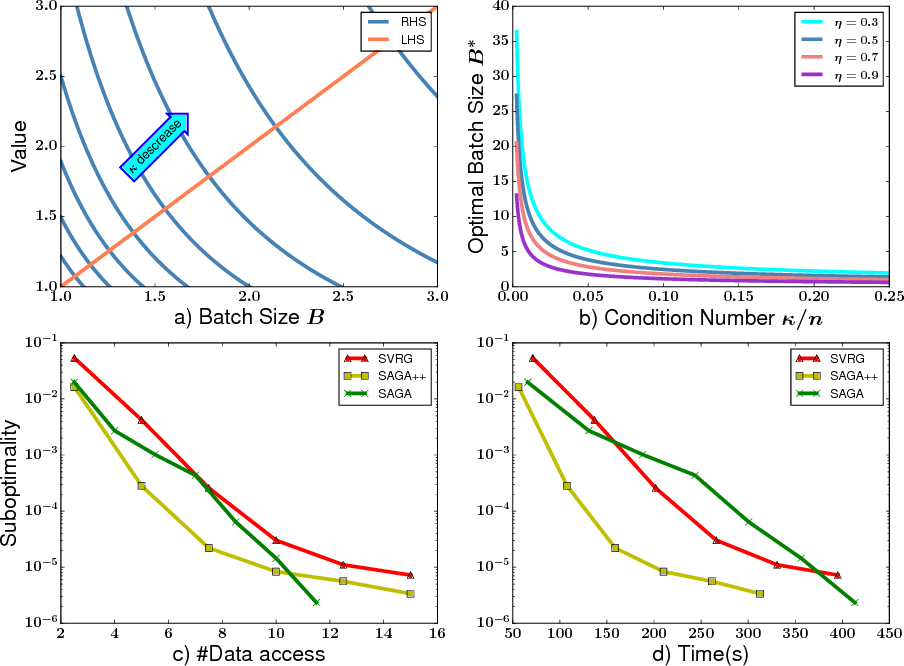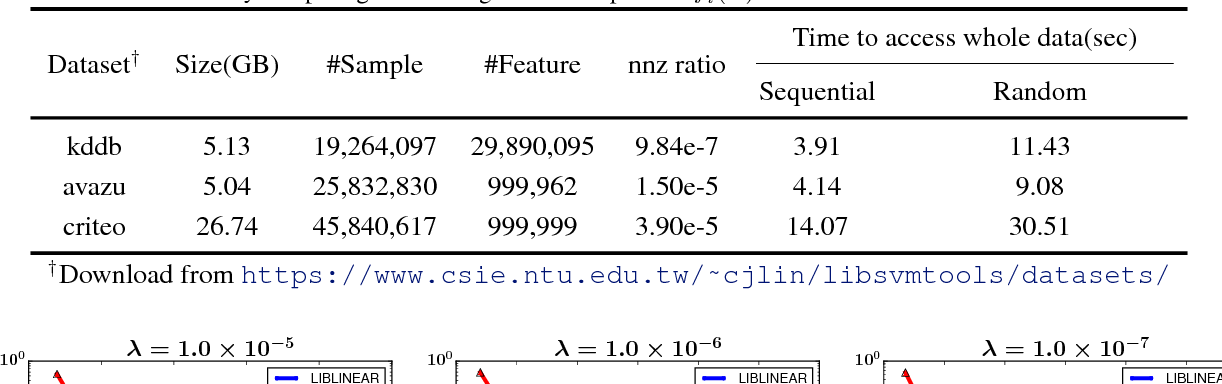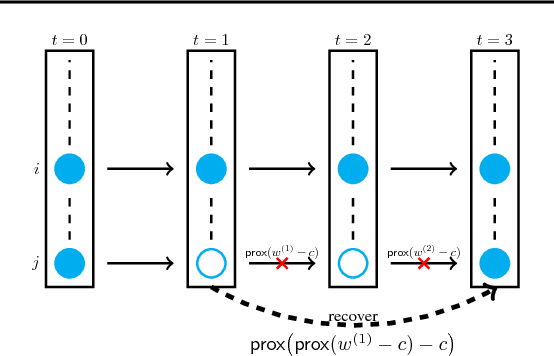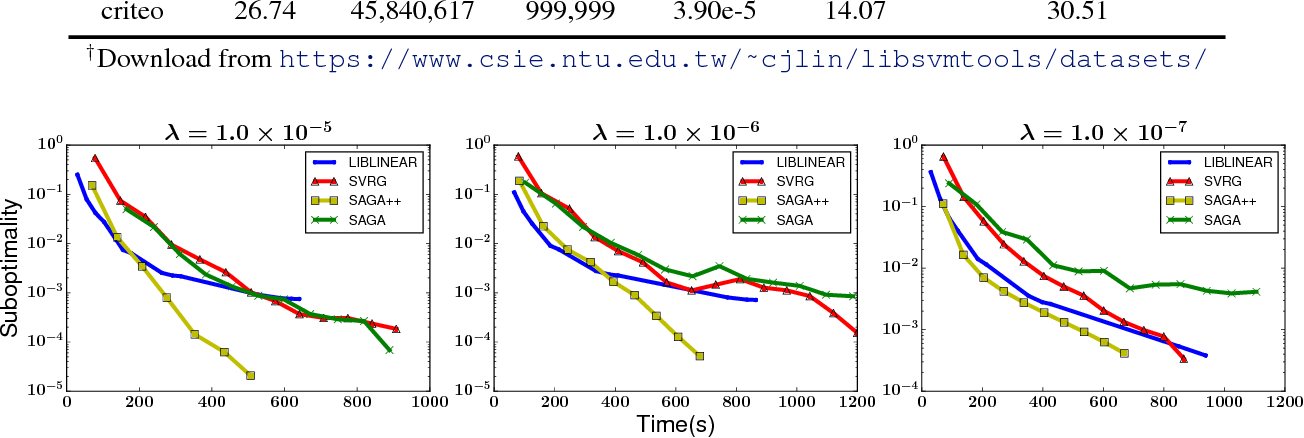In this paper we study a family of variance reduction methods with randomized batch size---at each step, the algorithm first randomly chooses the batch size and then selects a batch of samples to conduct a variance-reduced stochastic update. We give the linear convergence rate for this framework for composite functions, and show that the optimal strategy to achieve the optimal convergence rate per data access is to always choose batch size of 1, which is equivalent to the SAGA algorithm. However, due to the presence of cache/disk IO effect in computer architecture, the number of data access cannot reflect the running time because of 1) random memory access is much slower than sequential access, 2) when data is too big to fit into memory, disk seeking takes even longer time. After taking these into account, choosing batch size of $1$ is no longer optimal, so we propose a new algorithm called SAGA++ and show how to calculate the optimal average batch size theoretically. Our algorithm outperforms SAGA and other existing batched and stochastic solvers on real datasets. In addition, we also conduct a precise analysis to compare different update rules for variance reduction methods, showing that SAGA++ converges faster than SVRG in theory.

Click to Read Paper and Get Code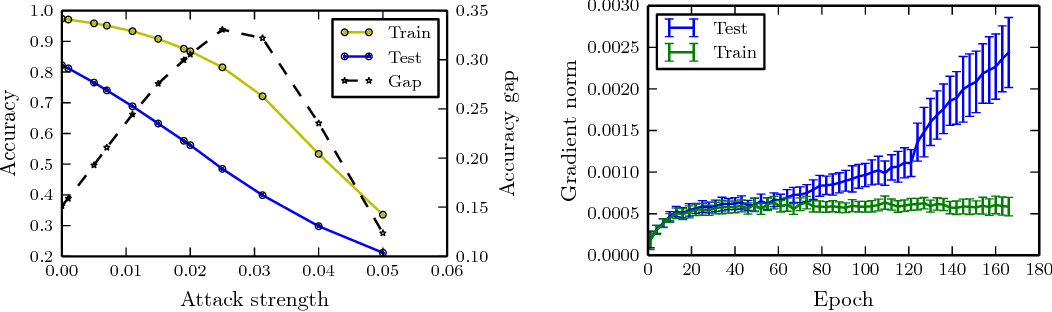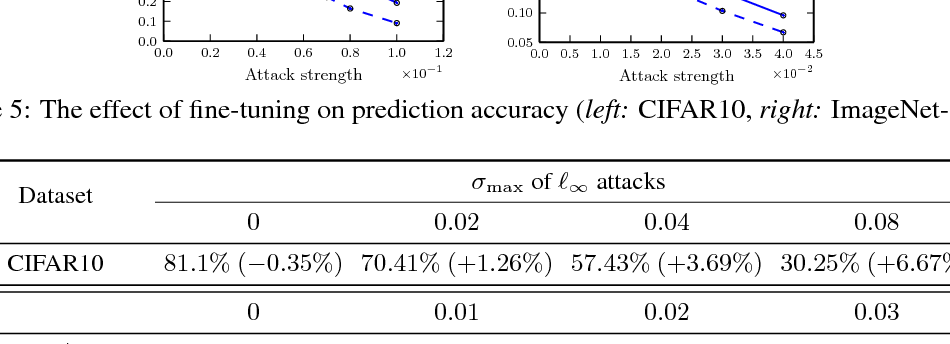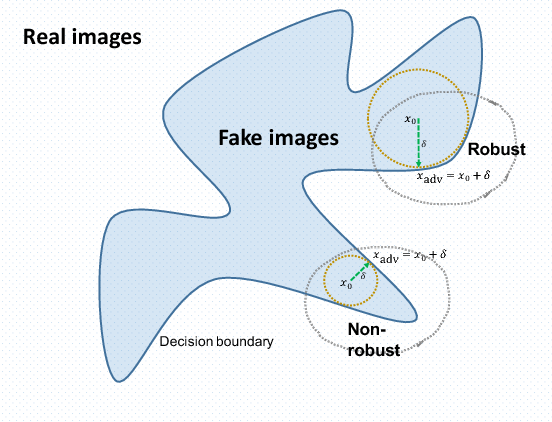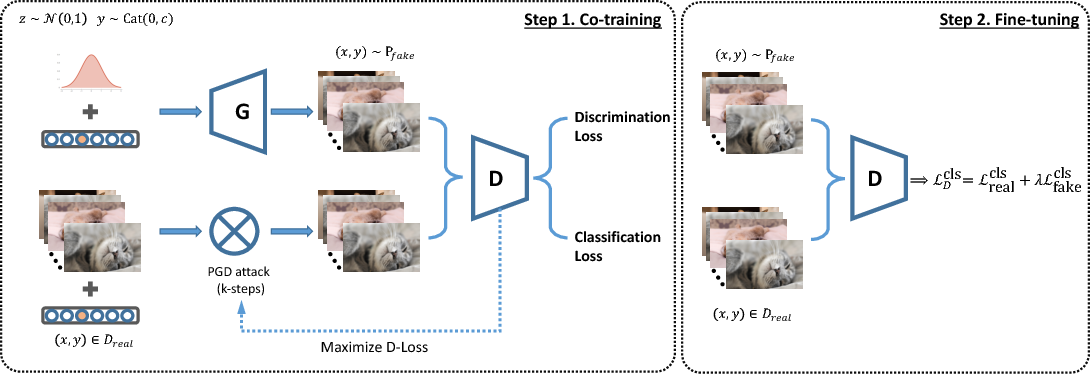In this paper, we are interested in two seemingly different concepts: \textit{adversarial training} and \textit{generative adversarial networks (GANs)}. Particularly, how these techniques help to improve each other. To this end, we analyze the limitation of adversarial training as the defense method, starting from questioning how well the robustness of a model can generalize. Then, we successfully improve the generalizability via data augmentation by the "fake" images sampled from generative adversarial networks. After that, we are surprised to see that the resulting robust classifier leads to a better generator, for free. We intuitively explain this interesting phenomenon and leave the theoretical analysis for future work. Motivated by these observations, we propose a system that combines generator, discriminator, and adversarial attacker in a single network. After end-to-end training and fine tuning, our method can simultaneously improve the robustness of classifiers, measured by accuracy under strong adversarial attacks; and the quality of generators, evaluated both aesthetically and quantitatively. In terms of the classifier, we achieve better robustness than the state-of-the-art adversarial training algorithm proposed in (Madry etla., 2017), while our generator achieves competitive performance compared with SN-GAN (Miyato and Koyama, 2018). Source code is publicly available online at \url{https://github.com/anonymous}.

* NIPS 2018 submission, under review. v2: More experiments on comparing inception score, release code and some minor fixes
Click to Read Paper and Get Code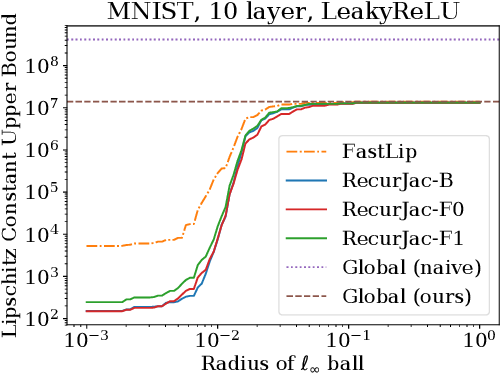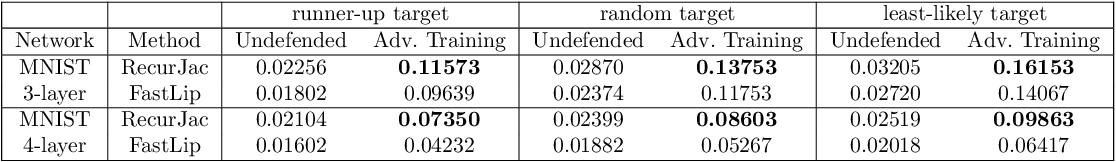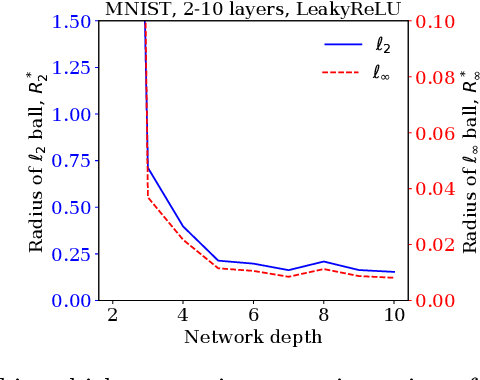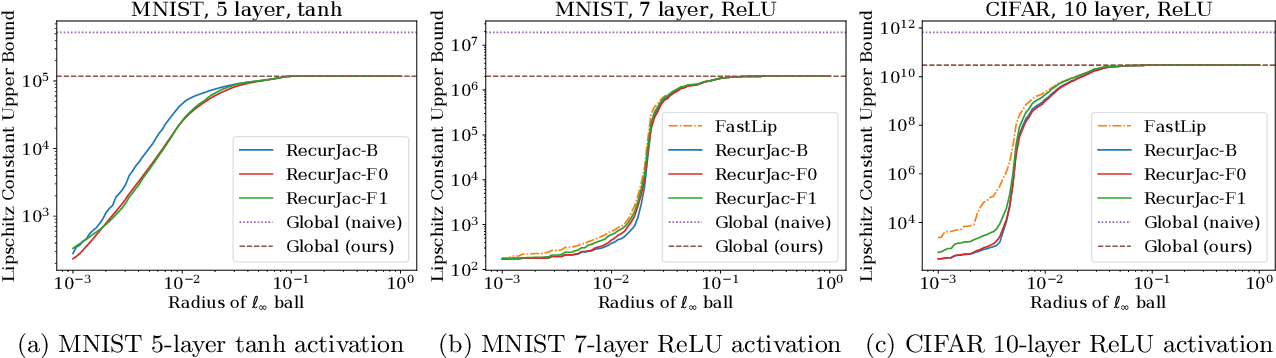The Jacobian matrix (or the gradient for single-output networks) is directly related to many important properties of neural networks, such as the function landscape, stationary points, (local) Lipschitz constants and robustness to adversarial attacks. In this paper, we propose a recursive algorithm, RecurJac, to compute both upper and lower bounds for each element in the Jacobian matrix of a neural network with respect to network's input, and the network can contain a wide range of activation functions. As a byproduct, we can efficiently obtain a (local) Lipschitz constant, which plays a crucial role in neural network robustness verification, as well as the training stability of GANs. Experiments show that (local) Lipschitz constants produced by our method is of better quality than previous approaches, thus providing better robustness verification results. Our algorithm has polynomial time complexity, and its computation time is reasonable even for relatively large networks. Additionally, we use our bounds of Jacobian matrix to characterize the landscape of the neural network, for example, to determine whether there exist stationary points in a local neighborhood. Source code available at https://github.com/huanzhang12/RecurJac-Jacobian-Bounds.

* Work done during internship at Microsoft Research
Click to Read Paper and Get Code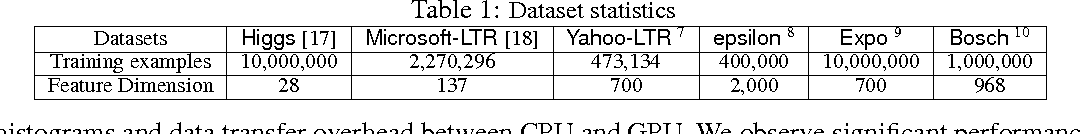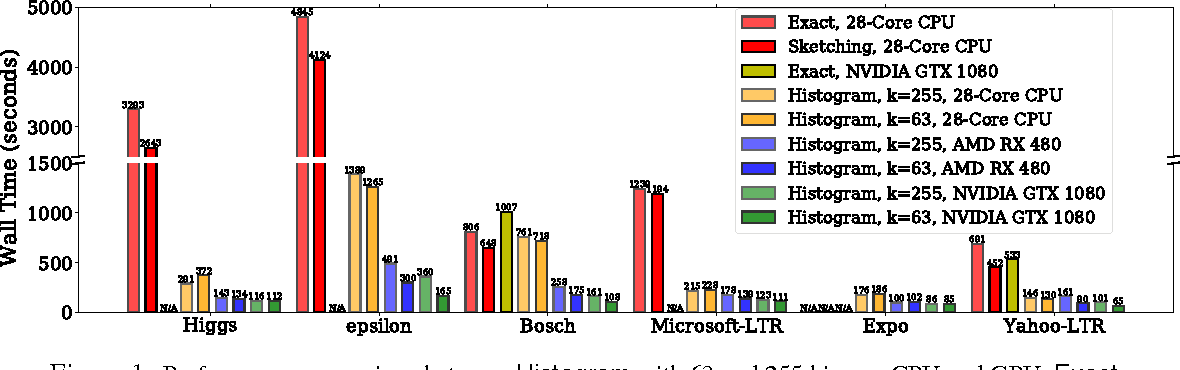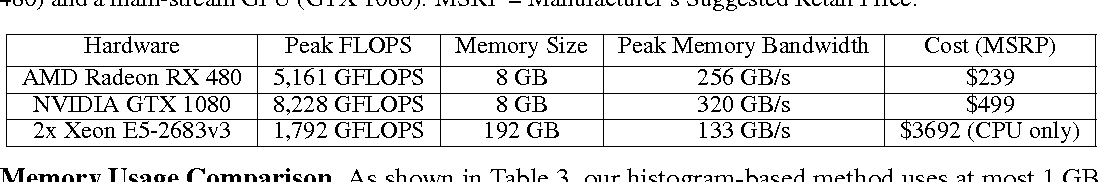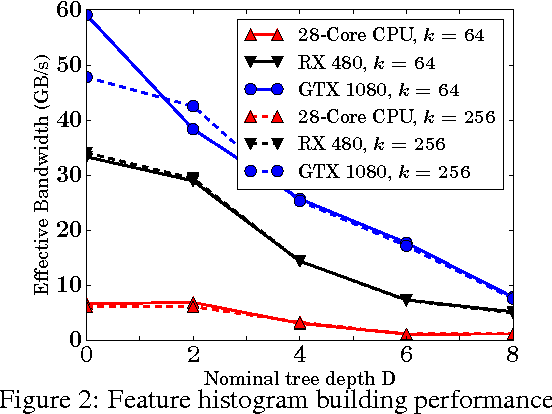In this paper, we present a novel massively parallel algorithm for accelerating the decision tree building procedure on GPUs (Graphics Processing Units), which is a crucial step in Gradient Boosted Decision Tree (GBDT) and random forests training. Previous GPU based tree building algorithms are based on parallel multi-scan or radix sort to find the exact tree split, and thus suffer from scalability and performance issues. We show that using a histogram based algorithm to approximately find the best split is more efficient and scalable on GPU. By identifying the difference between classical GPU-based image histogram construction and the feature histogram construction in decision tree training, we develop a fast feature histogram building kernel on GPU with carefully designed computational and memory access sequence to reduce atomic update conflict and maximize GPU utilization. Our algorithm can be used as a drop-in replacement for histogram construction in popular tree boosting systems to improve their scalability. As an example, to train GBDT on epsilon dataset, our method using a main-stream GPU is 7-8 times faster than histogram based algorithm on CPU in LightGBM and 25 times faster than the exact-split finding algorithm in XGBoost on a dual-socket 28-core Xeon server, while achieving similar prediction accuracy.

Click to Read Paper and Get Code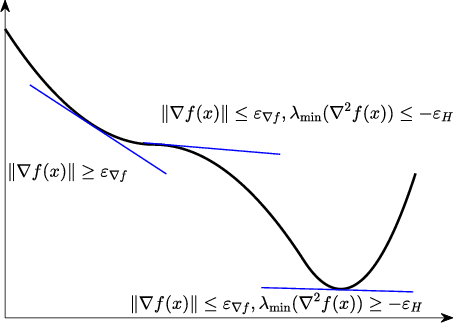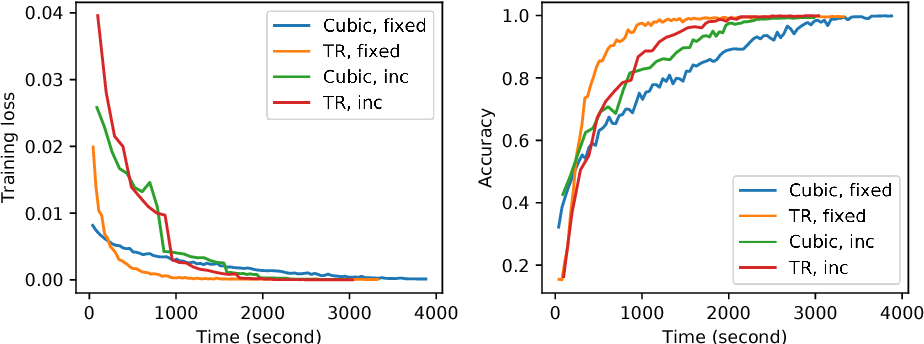Trust region and cubic regularization methods have demonstrated good performance in small scale non-convex optimization, showing the ability to escape from saddle points. Each iteration of these methods involves computation of gradient, Hessian and function value in order to obtain the search direction and adjust the radius or cubic regularization parameter. However, exactly computing those quantities are too expensive in large-scale problems such as training deep networks. In this paper, we study a family of stochastic trust region and cubic regularization methods when gradient, Hessian and function values are computed inexactly, and show the iteration complexity to achieve $\epsilon$-approximate second-order optimality is in the same order with previous work for which gradient and function values are computed exactly. The mild conditions on inexactness can be achieved in finite-sum minimization using random sampling. We show the algorithm performs well on training convolutional neural networks compared with previous second-order methods.

Click to Read Paper and Get Code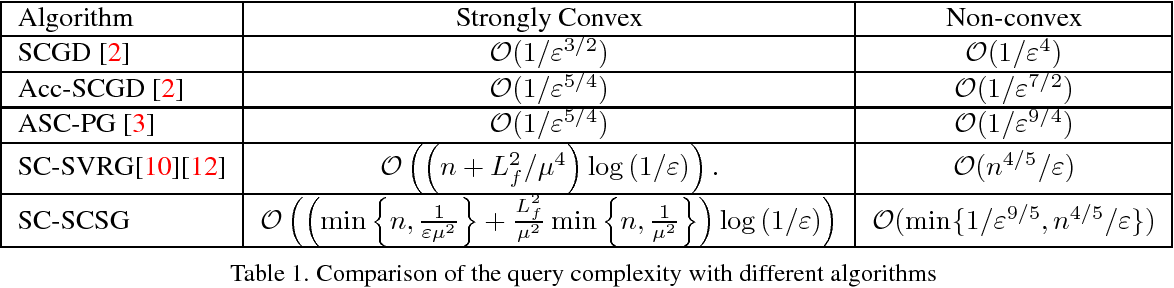In this paper, we consider the convex and non-convex composition problem with the structure $\frac{1}{n}\sum\nolimits_{i = 1}^n {{F_i}( {G( x )} )}$, where $G( x )=\frac{1}{n}\sum\nolimits_{j = 1}^n {{G_j}( x )}$ is the inner function, and $F_i(\cdot)$ is the outer function. We explore the variance reduction based method to solve the composition optimization. Due to the fact that when the number of inner function and outer function are large, it is not reasonable to estimate them directly, thus we apply the stochastically controlled stochastic gradient (SCSG) method to estimate the gradient of the composition function and the value of the inner function. The query complexity of our proposed method for the convex and non-convex problem is equal to or better than the current method for the composition problem. Furthermore, we also present the mini-batch version of the proposed method, which has the improved the query complexity with related to the size of the mini-batch.

Click to Read Paper and Get Code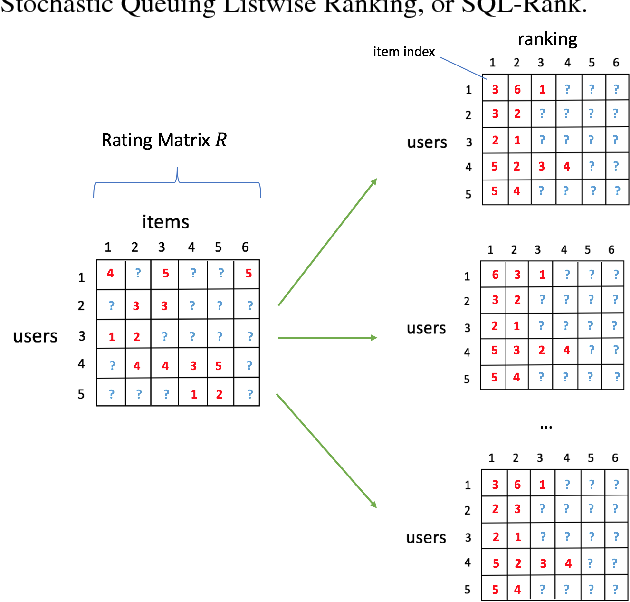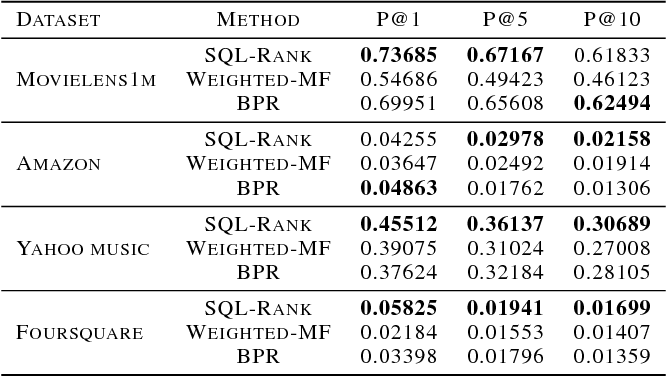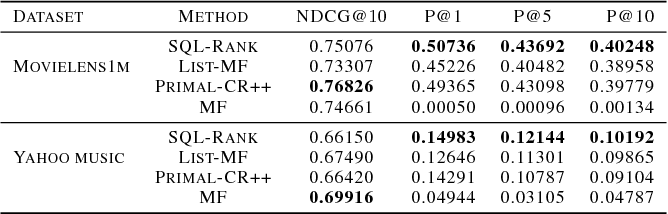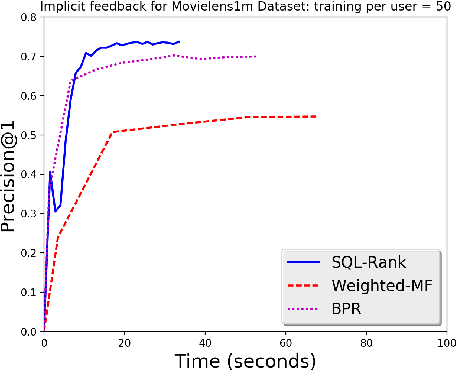In this paper, we propose a listwise approach for constructing user-specific rankings in recommendation systems in a collaborative fashion. We contrast the listwise approach to previous pointwise and pairwise approaches, which are based on treating either each rating or each pairwise comparison as an independent instance respectively. By extending the work of (Cao et al. 2007), we cast listwise collaborative ranking as maximum likelihood under a permutation model which applies probability mass to permutations based on a low rank latent score matrix. We present a novel algorithm called SQL-Rank, which can accommodate ties and missing data and can run in linear time. We develop a theoretical framework for analyzing listwise ranking methods based on a novel representation theory for the permutation model. Applying this framework to collaborative ranking, we derive asymptotic statistical rates as the number of users and items grow together. We conclude by demonstrating that our SQL-Rank method often outperforms current state-of-the-art algorithms for implicit feedback such as Weighted-MF and BPR and achieve favorable results when compared to explicit feedback algorithms such as matrix factorization and collaborative ranking.

Click to Read Paper and Get Code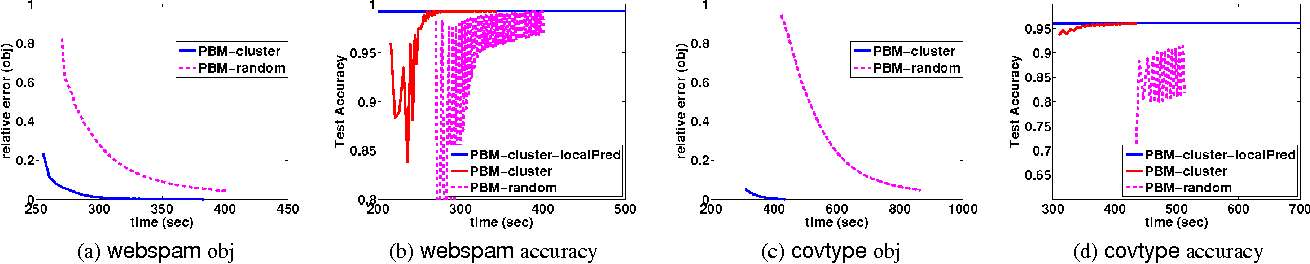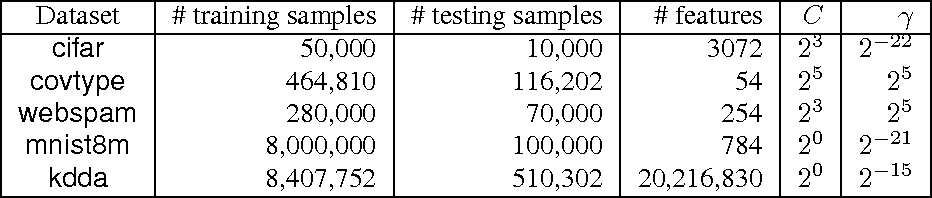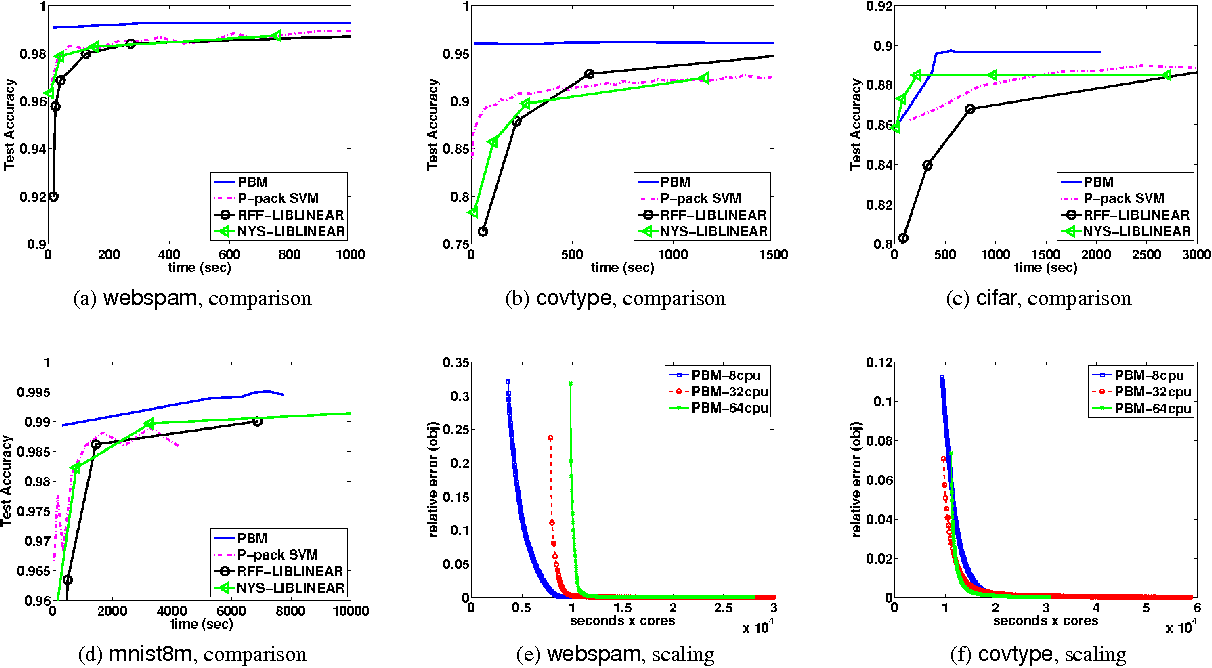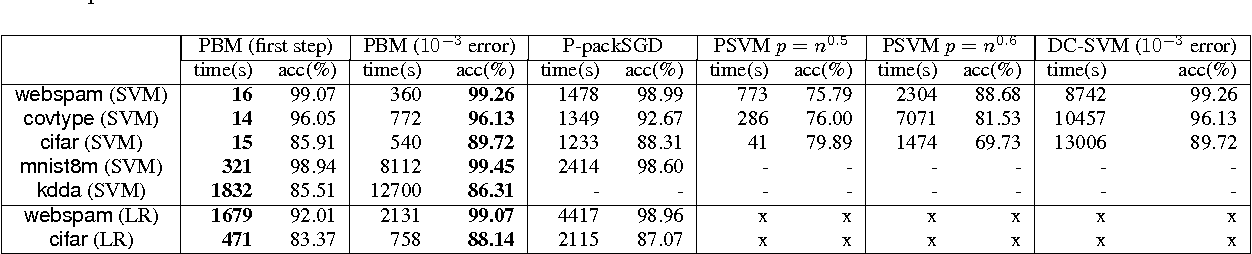Kernel machines often yield superior predictive performance on various tasks; however, they suffer from severe computational challenges. In this paper, we show how to overcome the important challenge of speeding up kernel machines. In particular, we develop a parallel block minimization framework for solving kernel machines, including kernel SVM and kernel logistic regression. Our framework proceeds by dividing the problem into smaller subproblems by forming a block-diagonal approximation of the Hessian matrix. The subproblems are then solved approximately in parallel. After that, a communication efficient line search procedure is developed to ensure sufficient reduction of the objective function value at each iteration. We prove global linear convergence rate of the proposed method with a wide class of subproblem solvers, and our analysis covers strongly convex and some non-strongly convex functions. We apply our algorithm to solve large-scale kernel SVM problems on distributed systems, and show a significant improvement over existing parallel solvers. As an example, on the covtype dataset with half-a-million samples, our algorithm can obtain an approximate solution with 96% accuracy in 20 seconds using 32 machines, while all the other parallel kernel SVM solvers require more than 2000 seconds to achieve a solution with 95% accuracy. Moreover, our algorithm can scale to very large data sets, such as the kdd algebra dataset with 8 million samples and 20 million features.

Click to Read Paper and Get Code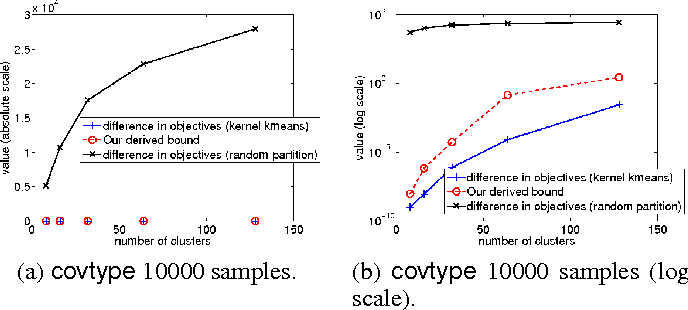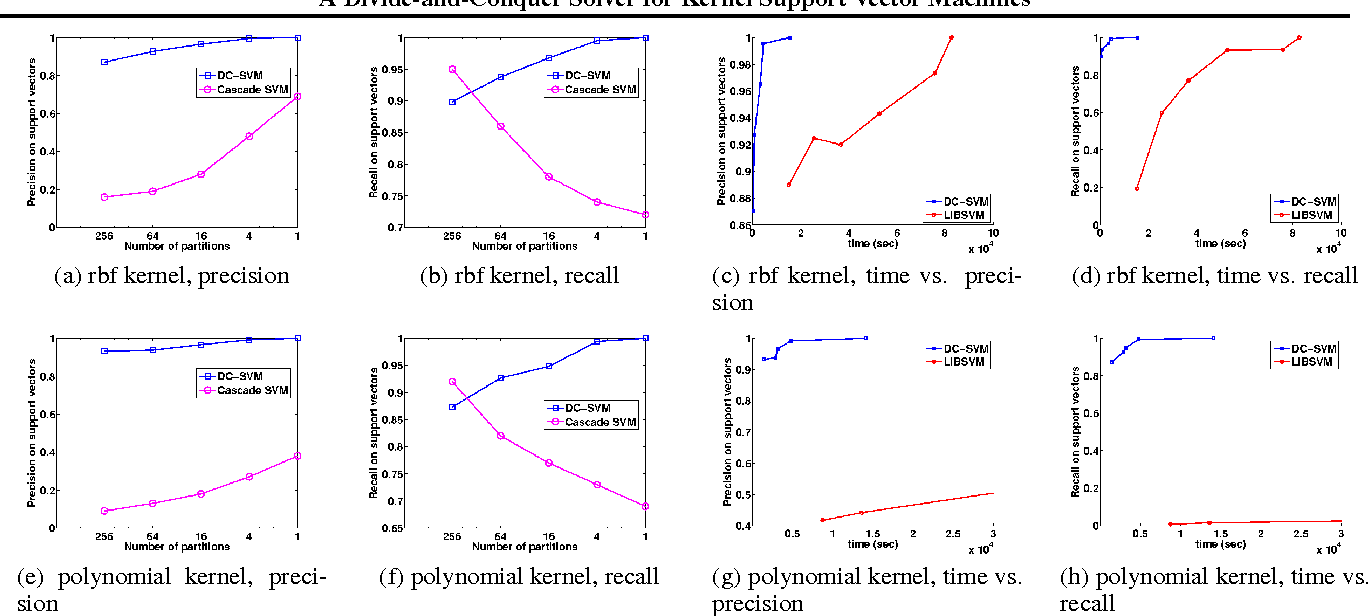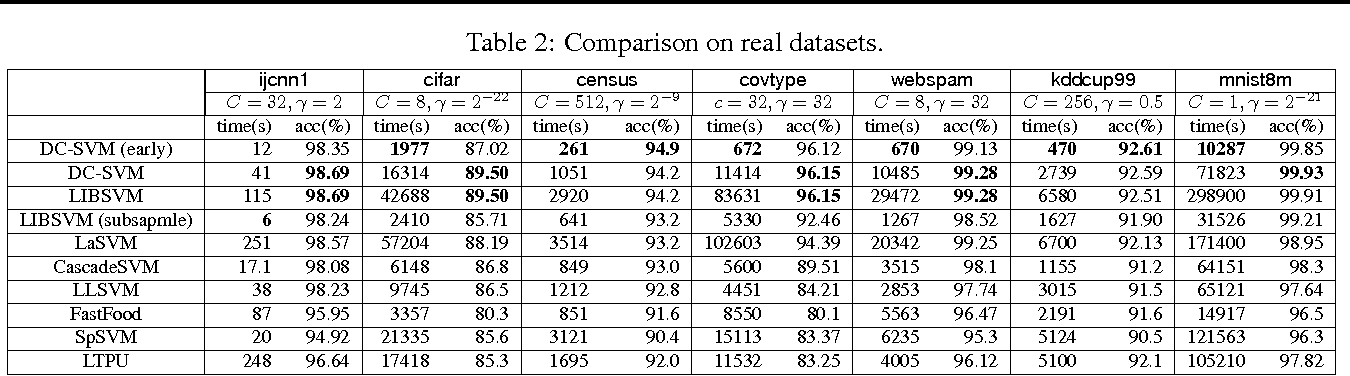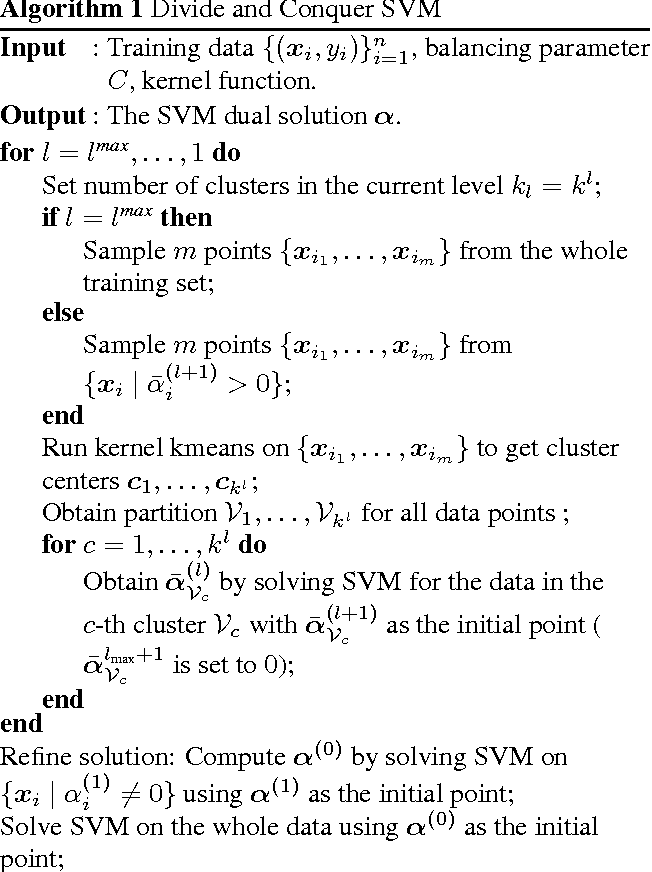The kernel support vector machine (SVM) is one of the most widely used classification methods; however, the amount of computation required becomes the bottleneck when facing millions of samples. In this paper, we propose and analyze a novel divide-and-conquer solver for kernel SVMs (DC-SVM). In the division step, we partition the kernel SVM problem into smaller subproblems by clustering the data, so that each subproblem can be solved independently and efficiently. We show theoretically that the support vectors identified by the subproblem solution are likely to be support vectors of the entire kernel SVM problem, provided that the problem is partitioned appropriately by kernel clustering. In the conquer step, the local solutions from the subproblems are used to initialize a global coordinate descent solver, which converges quickly as suggested by our analysis. By extending this idea, we develop a multilevel Divide-and-Conquer SVM algorithm with adaptive clustering and early prediction strategy, which outperforms state-of-the-art methods in terms of training speed, testing accuracy, and memory usage. As an example, on the covtype dataset with half-a-million samples, DC-SVM is 7 times faster than LIBSVM in obtaining the exact SVM solution (to within $10^{-6}$ relative error) which achieves 96.15% prediction accuracy. Moreover, with our proposed early prediction strategy, DC-SVM achieves about 96% accuracy in only 12 minutes, which is more than 100 times faster than LIBSVM.

Click to Read Paper and Get Code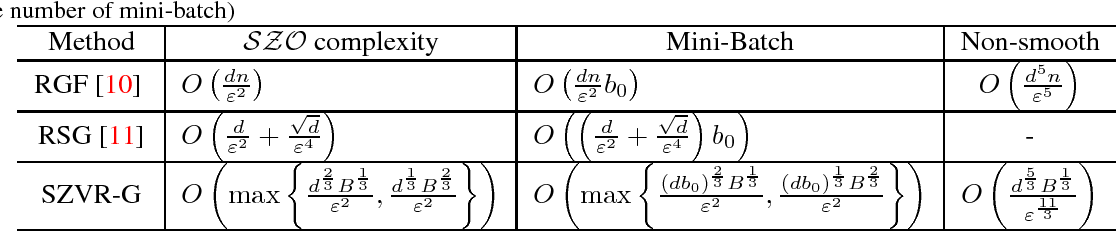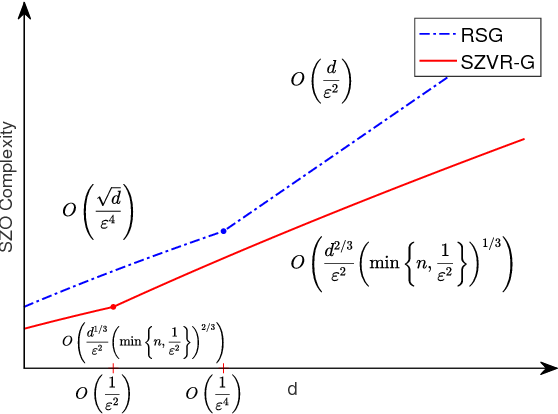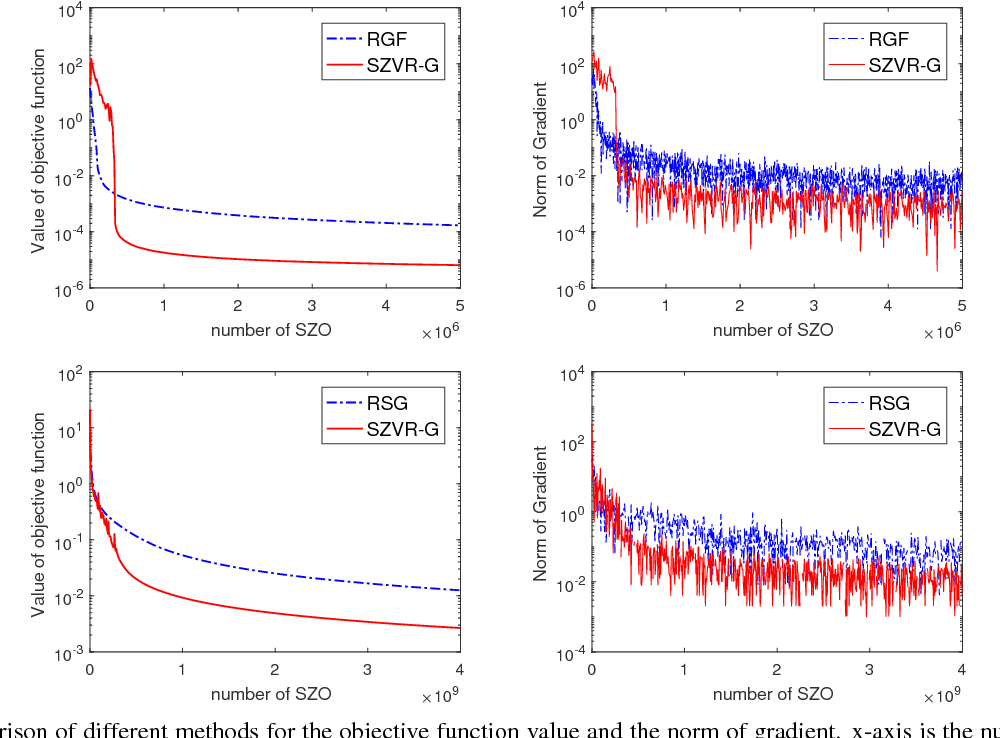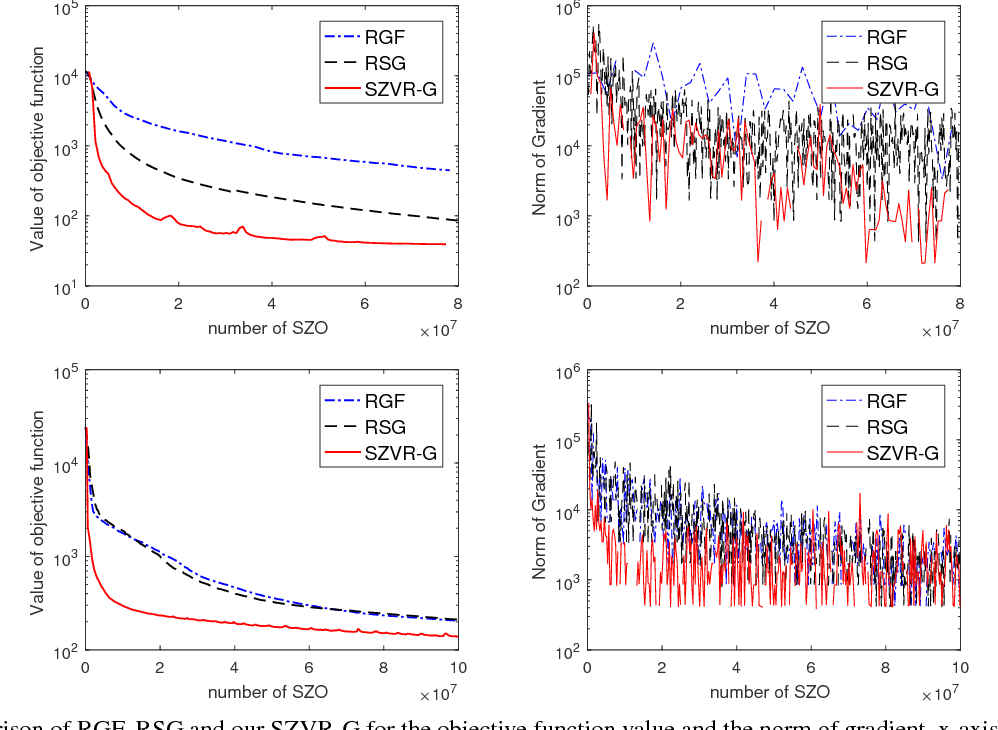Derivative-free optimization has become an important technique used in machine learning for optimizing black-box models. To conduct updates without explicitly computing gradient, most current approaches iteratively sample a random search direction from Gaussian distribution and compute the estimated gradient along that direction. However, due to the variance in the search direction, the convergence rates and query complexities of existing methods suffer from a factor of $d$, where $d$ is the problem dimension. In this paper, we introduce a novel Stochastic Zeroth-order method with Variance Reduction under Gaussian smoothing (SZVR-G) and establish the complexity for optimizing non-convex problems. With variance reduction on both sample space and search space, the complexity of our algorithm is sublinear to $d$ and is strictly better than current approaches, in both smooth and non-smooth cases. Moreover, we extend the proposed method to the mini-batch version. Our experimental results demonstrate the superior performance of the proposed method over existing derivative-free optimization techniques. Furthermore, we successfully apply our method to conduct a universal black-box attack to deep neural networks and present some interesting results.

Click to Read Paper and Get Code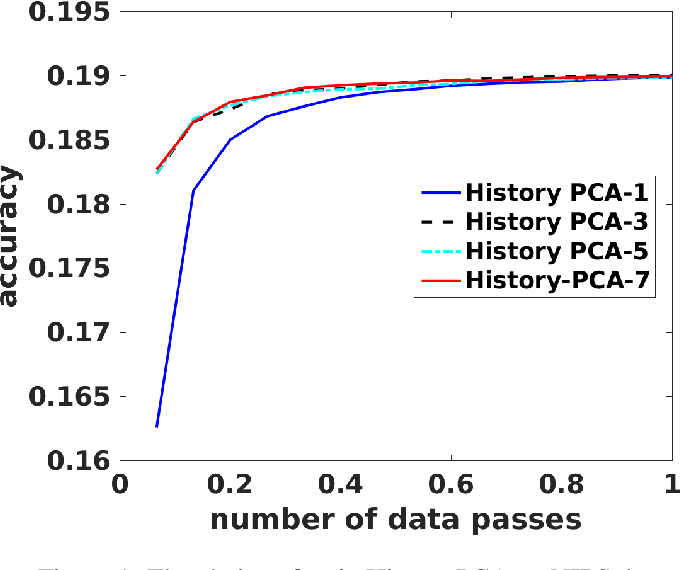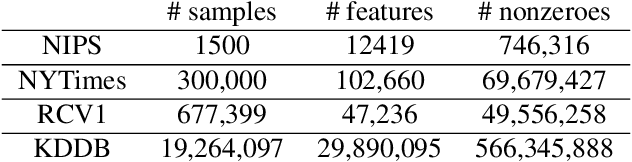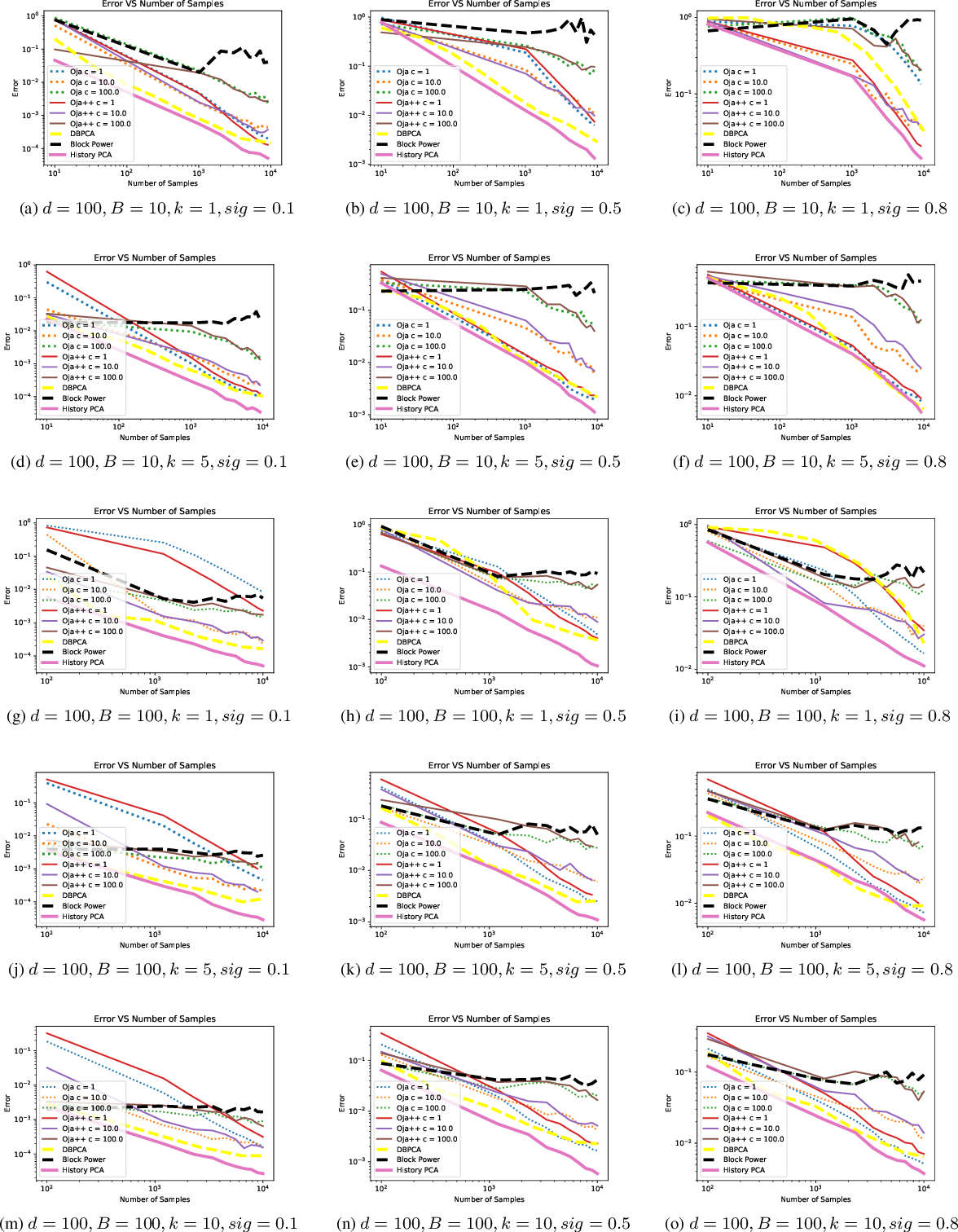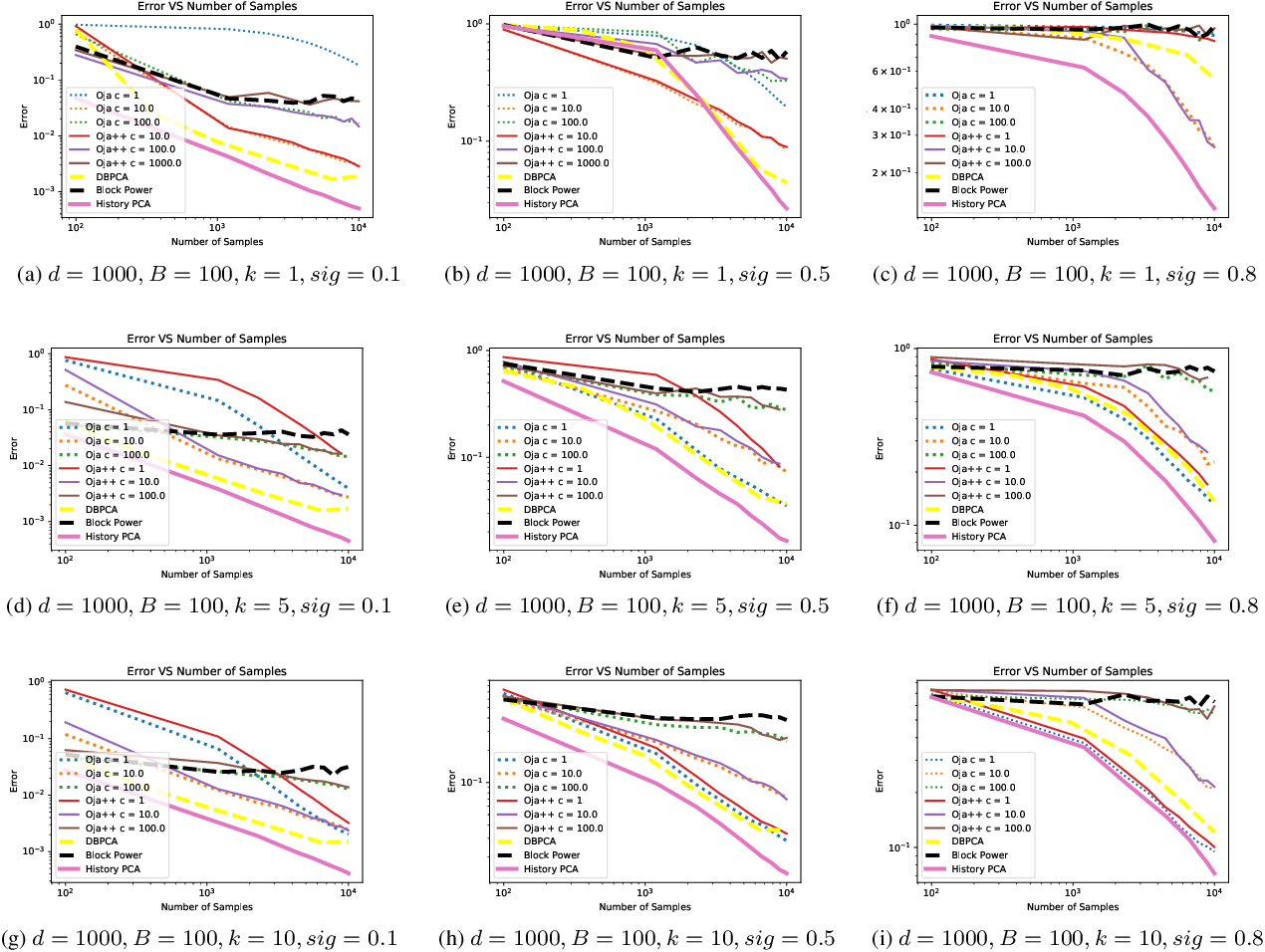In this paper we propose a new algorithm for streaming principal component analysis. With limited memory, small devices cannot store all the samples in the high-dimensional regime. Streaming principal component analysis aims to find the $k$-dimensional subspace which can explain the most variation of the $d$-dimensional data points that come into memory sequentially. In order to deal with large $d$ and large $N$ (number of samples), most streaming PCA algorithms update the current model using only the incoming sample and then dump the information right away to save memory. However the information contained in previously streamed data could be useful. Motivated by this idea, we develop a new streaming PCA algorithm called History PCA that achieves this goal. By using $O(Bd)$ memory with $B\approx 10$ being the block size, our algorithm converges much faster than existing streaming PCA algorithms. By changing the number of inner iterations, the memory usage can be further reduced to $O(d)$ while maintaining a comparable convergence speed. We provide theoretical guarantees for the convergence of our algorithm along with the rate of convergence. We also demonstrate on synthetic and real world data sets that our algorithm compares favorably with other state-of-the-art streaming PCA methods in terms of the convergence speed and performance.

Click to Read Paper and Get Code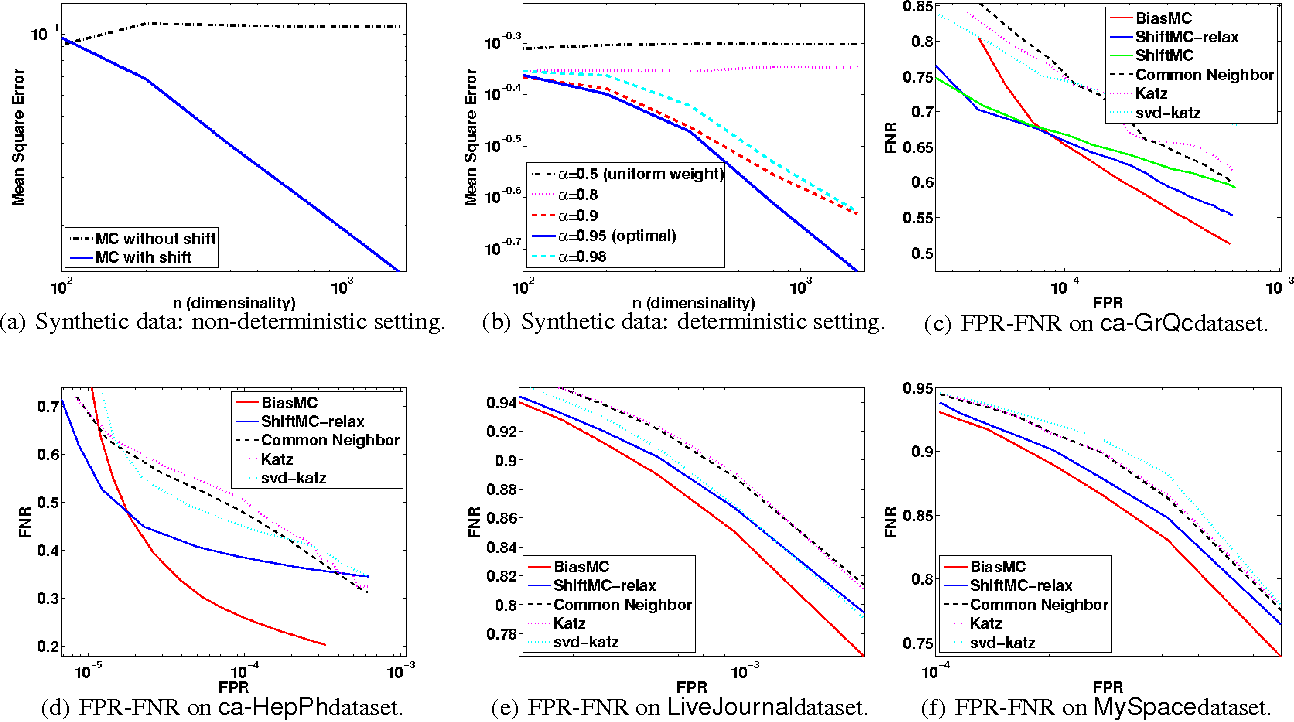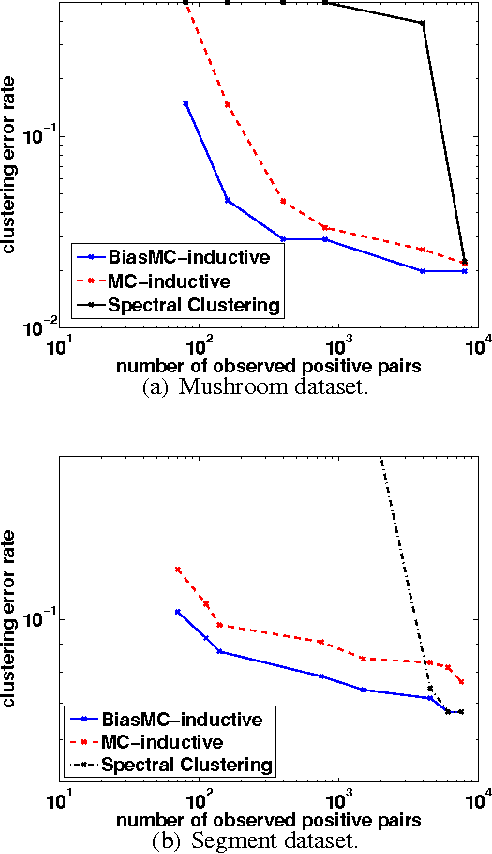In this paper, we consider the matrix completion problem when the observations are one-bit measurements of some underlying matrix M, and in particular the observed samples consist only of ones and no zeros. This problem is motivated by modern applications such as recommender systems and social networks where only "likes" or "friendships" are observed. The problem of learning from only positive and unlabeled examples, called PU (positive-unlabeled) learning, has been studied in the context of binary classification. We consider the PU matrix completion problem, where an underlying real-valued matrix M is first quantized to generate one-bit observations and then a subset of positive entries is revealed. Under the assumption that M has bounded nuclear norm, we provide recovery guarantees for two different observation models: 1) M parameterizes a distribution that generates a binary matrix, 2) M is thresholded to obtain a binary matrix. For the first case, we propose a "shifted matrix completion" method that recovers M using only a subset of indices corresponding to ones, while for the second case, we propose a "biased matrix completion" method that recovers the (thresholded) binary matrix. Both methods yield strong error bounds --- if M is n by n, the Frobenius error is bounded as O(1/((1-rho)n), where 1-rho denotes the fraction of ones observed. This implies a sample complexity of O(n\log n) ones to achieve a small error, when M is dense and n is large. We extend our methods and guarantees to the inductive matrix completion problem, where rows and columns of M have associated features. We provide efficient and scalable optimization procedures for both the methods and demonstrate the effectiveness of the proposed methods for link prediction (on real-world networks consisting of over 2 million nodes and 90 million links) and semi-supervised clustering tasks.

Click to Read Paper and Get Code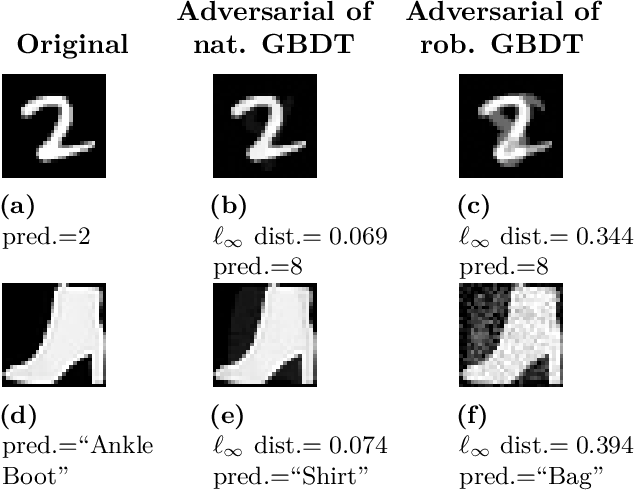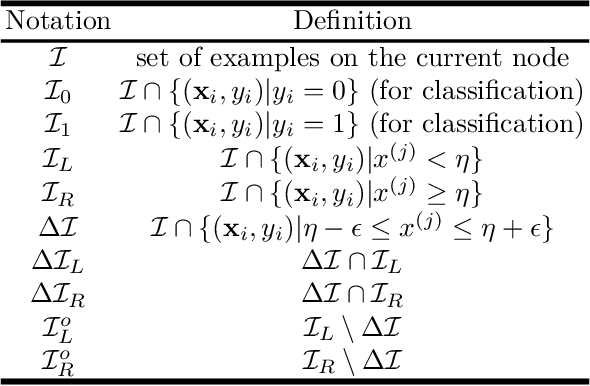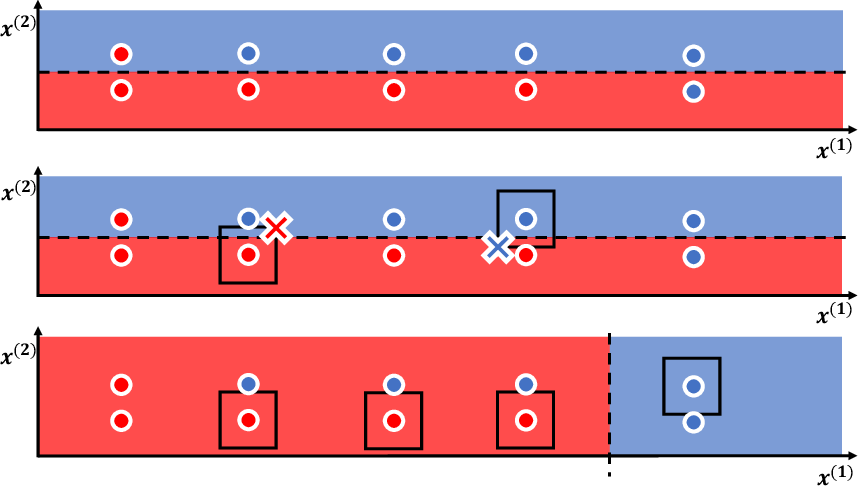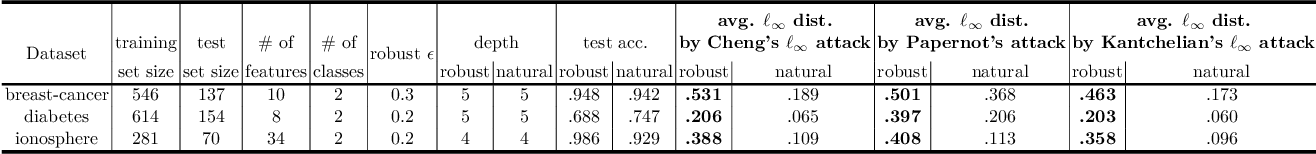Although adversarial examples and model robustness have been extensively studied in the context of linear models and neural networks, research on this issue in tree-based models and how to make tree-based models robust against adversarial examples is still limited. In this paper, we show that tree based models are also vulnerable to adversarial examples and develop a novel algorithm to learn robust trees. At its core, our method aims to optimize the performance under the worst-case perturbation of input features, which leads to a max-min saddle point problem. Incorporating this saddle point objective into the decision tree building procedure is non-trivial due to the discrete nature of trees --- a naive approach to finding the best split according to this saddle point objective will take exponential time. To make our approach practical and scalable, we propose efficient tree building algorithms by approximating the inner minimizer in this saddle point problem, and present efficient implementations for classical information gain based trees as well as state-of-the-art tree boosting models such as XGBoost. Experimental results on real world datasets demonstrate that the proposed algorithms can substantially improve the robustness of tree-based models against adversarial examples.

Click to Read Paper and Get Code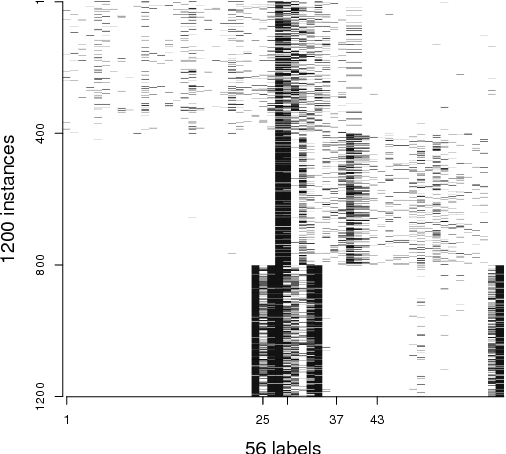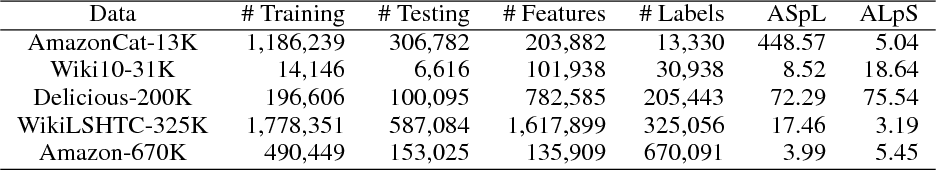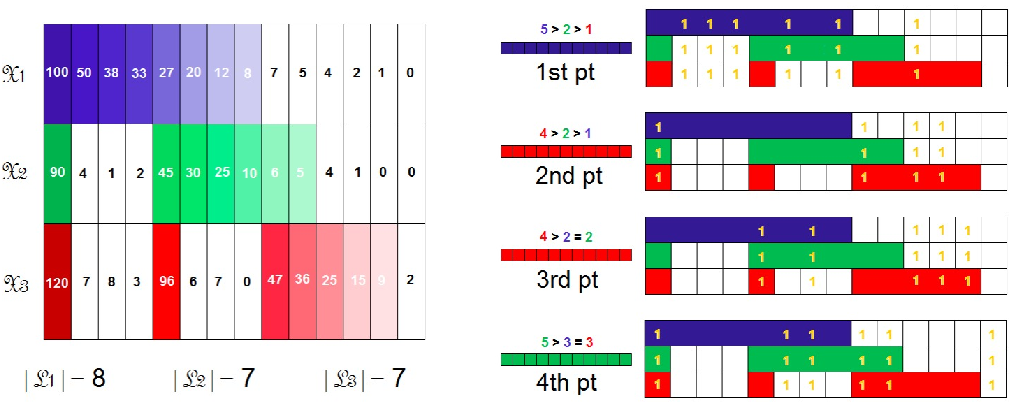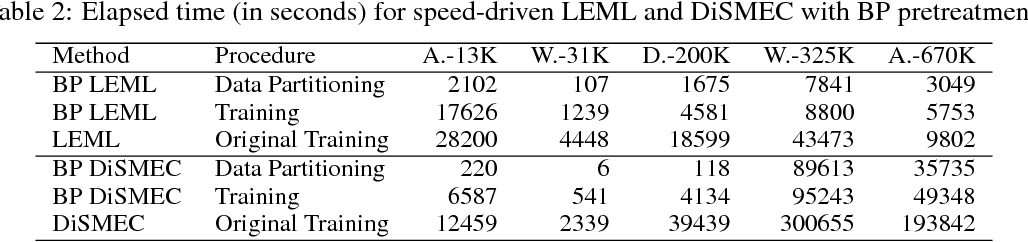Extreme multi-label classification aims to learn a classifier that annotates an instance with a relevant subset of labels from an extremely large label set. Many existing solutions embed the label matrix to a low-dimensional linear subspace, or examine the relevance of a test instance to every label via a linear scan. In practice, however, those approaches can be computationally exorbitant. To alleviate this drawback, we propose a Block-wise Partitioning (BP) pretreatment that divides all instances into disjoint clusters, to each of which the most frequently tagged label subset is attached. One multi-label classifier is trained on one pair of instance and label clusters, and the label set of a test instance is predicted by first delivering it to the most appropriate instance cluster. Experiments on benchmark multi-label data sets reveal that BP pretreatment significantly reduces prediction time, and retains almost the same level of prediction accuracy.

Click to Read Paper and Get Code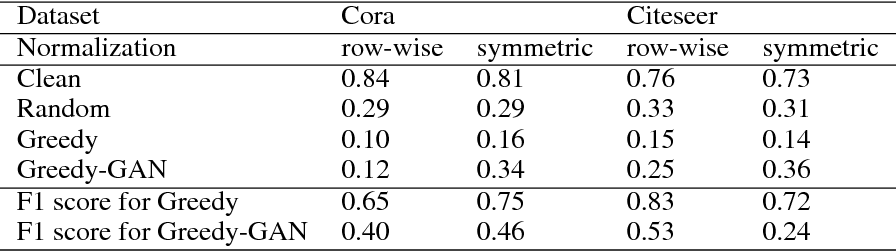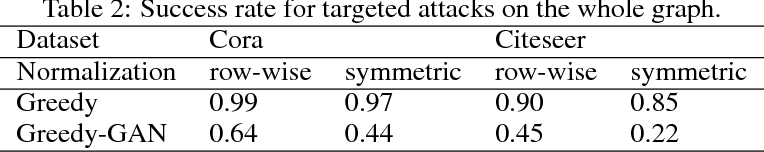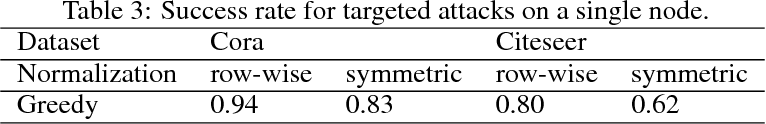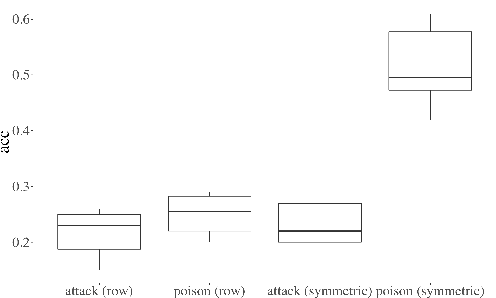Graph convolutional networks (GCNs) have been widely used for classifying graph nodes in the semi-supervised setting. Previous work have shown that GCNs are vulnerable to the perturbation on adjacency and feature matrices of existing nodes. However, it is unrealistic to change existing nodes in many applications, such as existing users in social networks. In this paper, we design algorithms to attack GCNs by adding fake nodes. A greedy algorithm is proposed to generate adjacency and feature matrices of fake nodes, aiming to minimize the classification accuracy on the existing nodes. In additional, we introduce a discriminator to classify fake nodes from real nodes, and propose a Greedy-GAN attack to simultaneously update the discriminator and the attacker, to make fake nodes indistinguishable to the real ones. Our non-targeted attack decreases the accuracy of GCN down to 0.10, and our targeted attack reaches a success rate of 99% on the whole datasets, and 94% on average for attacking a single target node.

Click to Read Paper and Get Code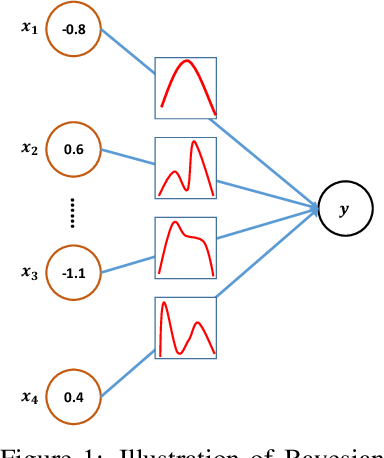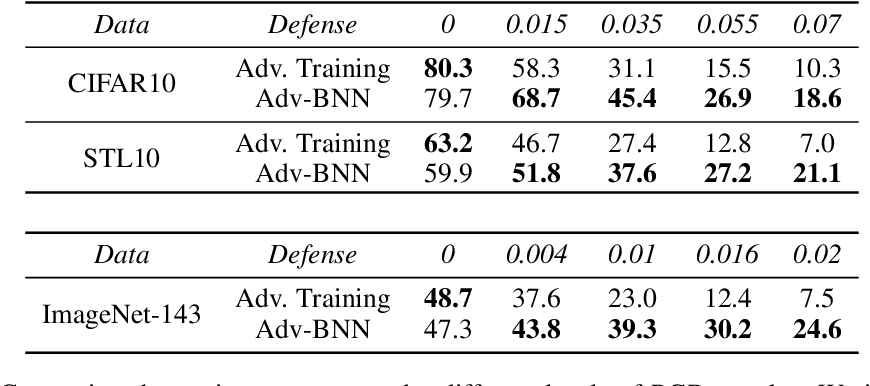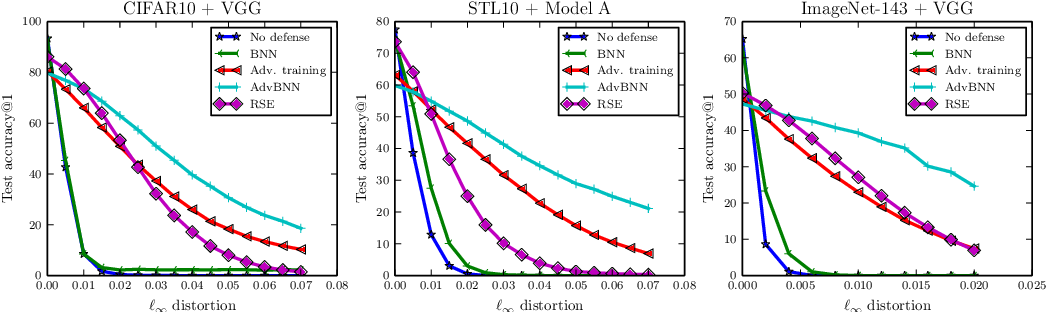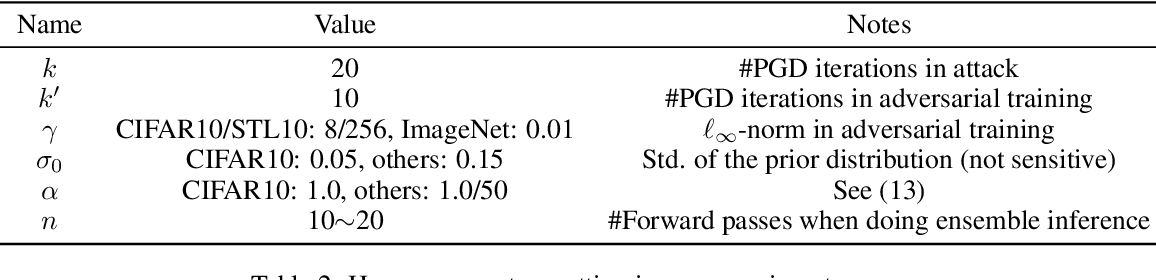We present a new algorithm to train a robust neural network against adversarial attacks. Our algorithm is motivated by the following two ideas. First, although recent work has demonstrated that fusing randomness can improve the robustness of neural networks (Liu 2017), we noticed that adding noise blindly to all the layers is not the optimal way to incorporate randomness. Instead, we model randomness under the framework of Bayesian Neural Network (BNN) to formally learn the posterior distribution of models in a scalable way. Second, we formulate the mini-max problem in BNN to learn the best model distribution under adversarial attacks, leading to an adversarial-trained Bayesian neural net. Experiment results demonstrate that the proposed algorithm achieves state-of-the-art performance under strong attacks. On CIFAR-10 with VGG network, our model leads to 14\% accuracy improvement compared with adversarial training (Madry 2017) and random self-ensemble (Liu 2017) under PGD attack with $0.035$ distortion, and the gap becomes even larger on a subset of ImageNet.

* Code will be made available at https://github.com/xuanqing94/BayesianDefense
Click to Read Paper and Get Code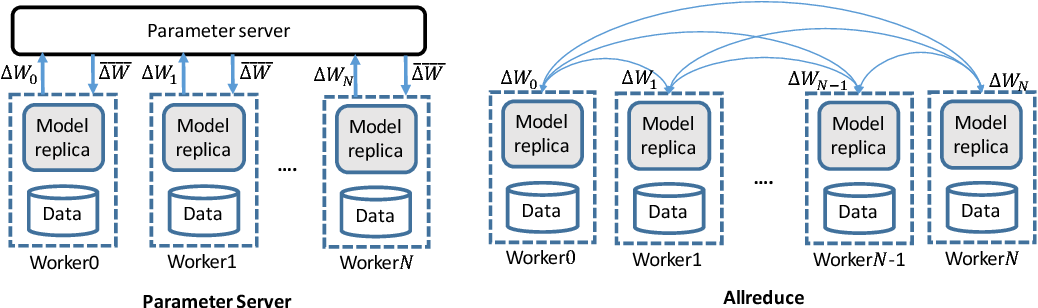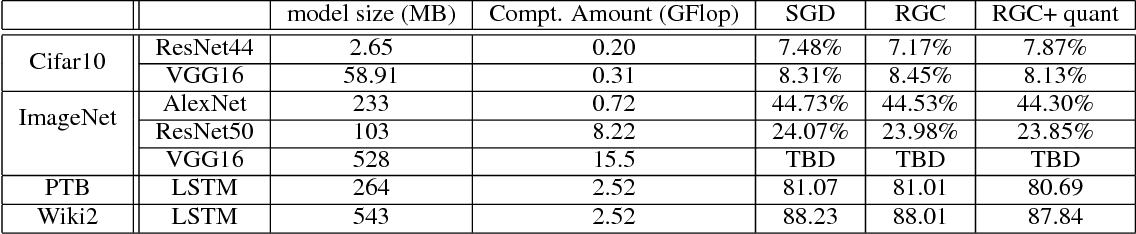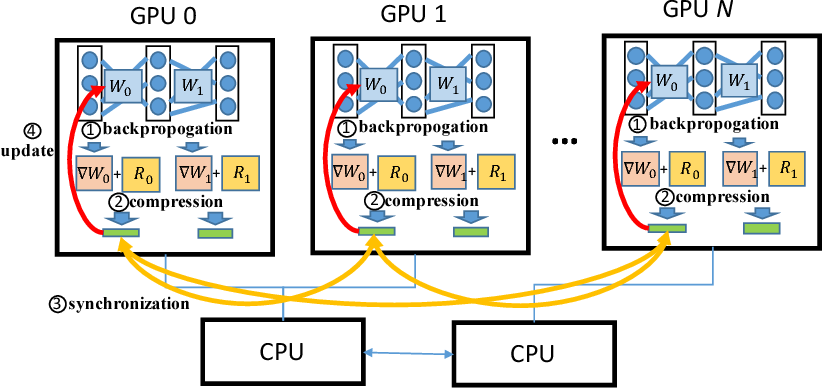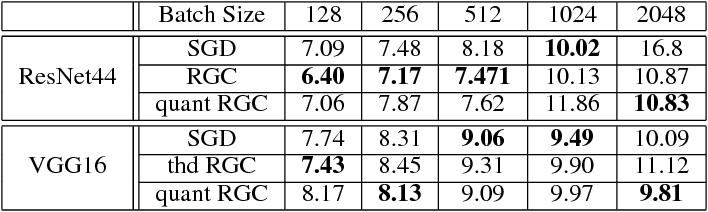Data parallelism has already become a dominant method to scale Deep Neural Network (DNN) training to multiple computation nodes. Considering that the synchronization of local model or gradient between iterations can be a bottleneck for large-scale distributed training, compressing communication traffic has gained widespread attention recently. Among several recent proposed compression algorithms, Residual Gradient Compression (RGC) is one of the most successful approaches---it can significantly compress the message size (0.1% of the original size) and still preserve accuracy. However, the literature on compressing deep networks focuses almost exclusively on finding good compression rate, while the efficiency of RGC in real implementation has been less investigated. In this paper, we explore the potential of application RGC method in the real distributed system. Targeting the widely adopted multi-GPU system, we proposed an RGC system design call RedSync, which includes a set of optimizations to reduce communication bandwidth while introducing limited overhead. We examine the performance of RedSync on two different multiple GPU platforms, including a supercomputer and a multi-card server. Our test cases include image classification and language modeling tasks on Cifar10, ImageNet, Penn Treebank and Wiki2 datasets. For DNNs featured with high communication to computation ratio, which have long been considered with poor scalability, RedSync shows significant performance improvement.

* 20 pages
Click to Read Paper and Get Code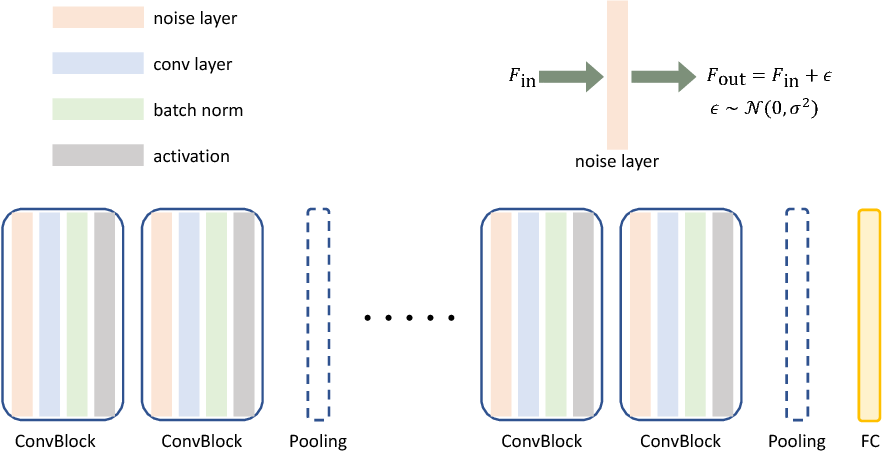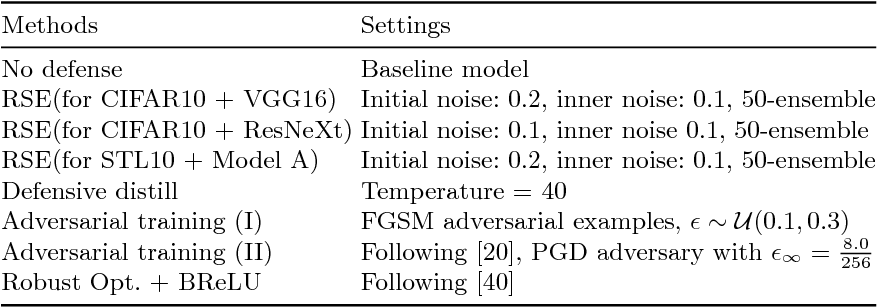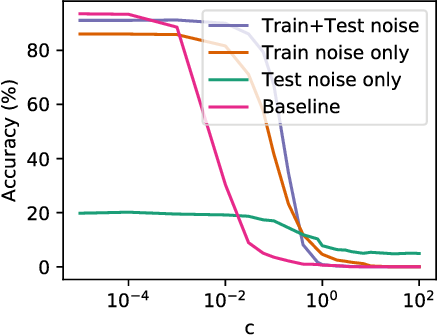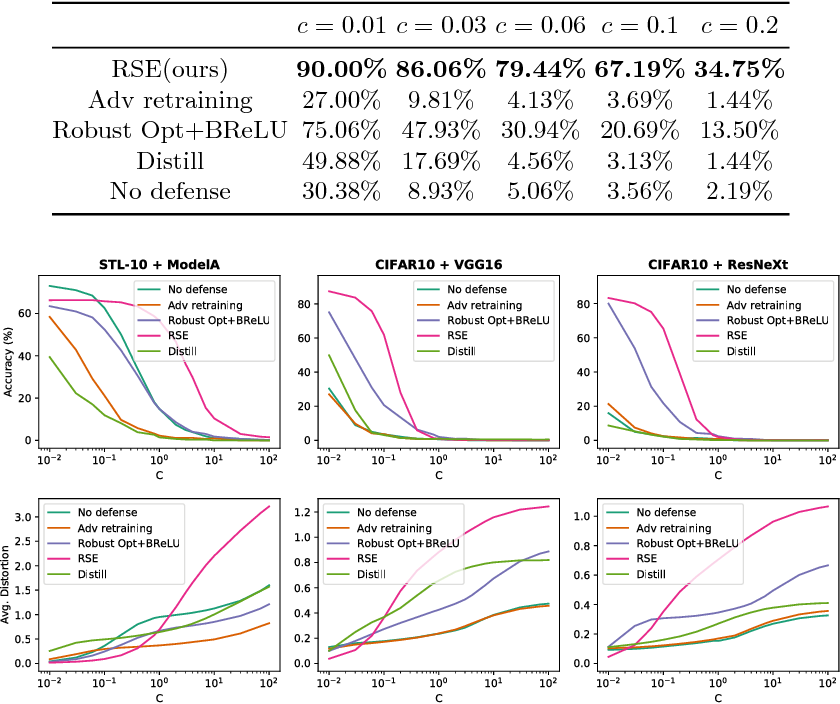Recent studies have revealed the vulnerability of deep neural networks: A small adversarial perturbation that is imperceptible to human can easily make a well-trained deep neural network misclassify. This makes it unsafe to apply neural networks in security-critical applications. In this paper, we propose a new defense algorithm called Random Self-Ensemble (RSE) by combining two important concepts: {\bf randomness} and {\bf ensemble}. To protect a targeted model, RSE adds random noise layers to the neural network to prevent the strong gradient-based attacks, and ensembles the prediction over random noises to stabilize the performance. We show that our algorithm is equivalent to ensemble an infinite number of noisy models $f_\epsilon$ without any additional memory overhead, and the proposed training procedure based on noisy stochastic gradient descent can ensure the ensemble model has a good predictive capability. Our algorithm significantly outperforms previous defense techniques on real data sets. For instance, on CIFAR-10 with VGG network (which has 92\% accuracy without any attack), under the strong C\&W attack within a certain distortion tolerance, the accuracy of unprotected model drops to less than 10\%, the best previous defense technique has $48\%$ accuracy, while our method still has $86\%$ prediction accuracy under the same level of attack. Finally, our method is simple and easy to integrate into any neural network.

* ECCV 2018 camera ready
Click to Read Paper and Get Code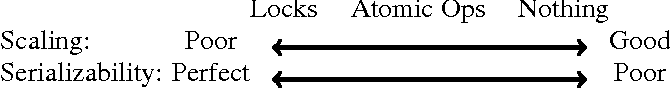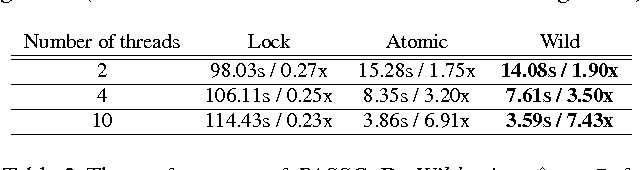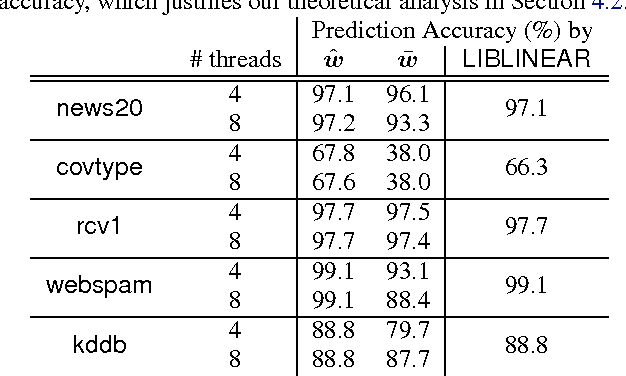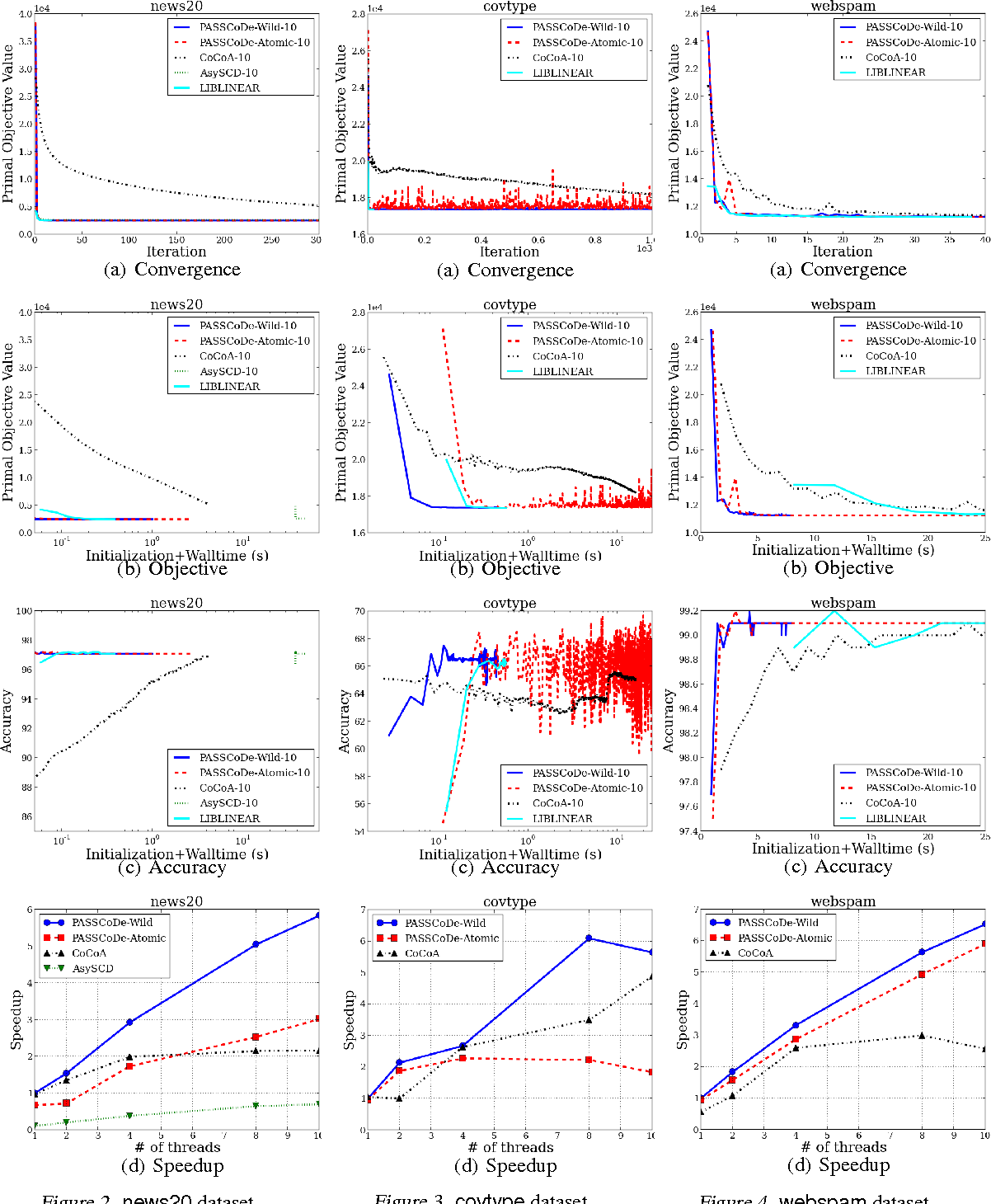Stochastic Dual Coordinate Descent (SDCD) has become one of the most efficient ways to solve the family of $\ell_2$-regularized empirical risk minimization problems, including linear SVM, logistic regression, and many others. The vanilla implementation of DCD is quite slow; however, by maintaining primal variables while updating dual variables, the time complexity of SDCD can be significantly reduced. Such a strategy forms the core algorithm in the widely-used LIBLINEAR package. In this paper, we parallelize the SDCD algorithms in LIBLINEAR. In recent research, several synchronized parallel SDCD algorithms have been proposed, however, they fail to achieve good speedup in the shared memory multi-core setting. In this paper, we propose a family of asynchronous stochastic dual coordinate descent algorithms (ASDCD). Each thread repeatedly selects a random dual variable and conducts coordinate updates using the primal variables that are stored in the shared memory. We analyze the convergence properties when different locking/atomic mechanisms are applied. For implementation with atomic operations, we show linear convergence under mild conditions. For implementation without any atomic operations or locking, we present the first {\it backward error analysis} for ASDCD under the multi-core environment, showing that the converged solution is the exact solution for a primal problem with perturbed regularizer. Experimental results show that our methods are much faster than previous parallel coordinate descent solvers.

Click to Read Paper and Get Code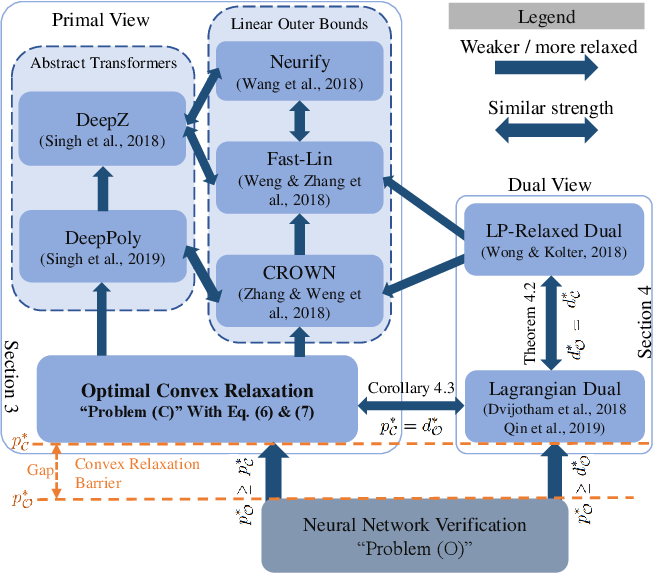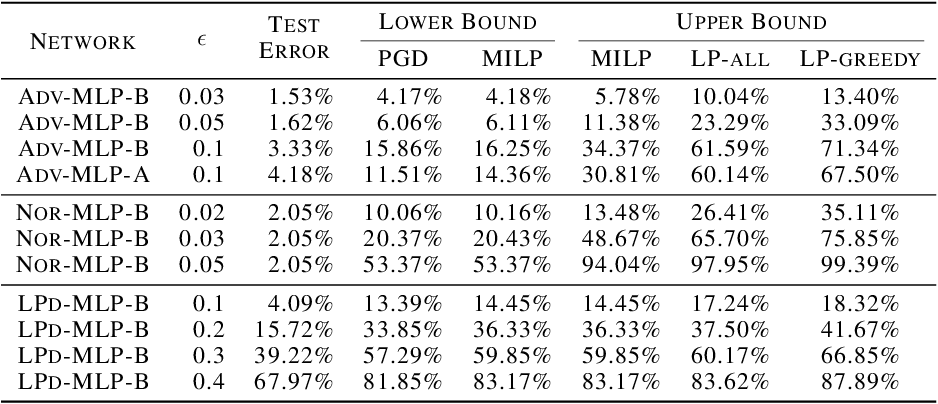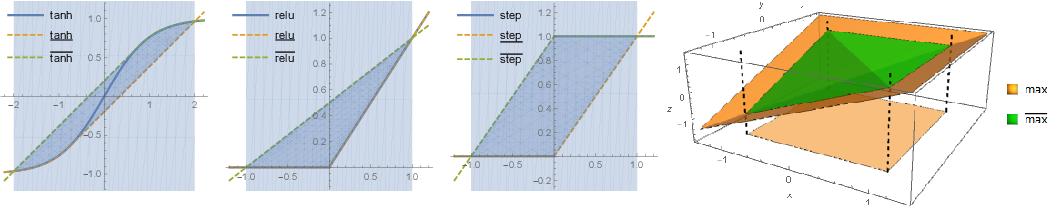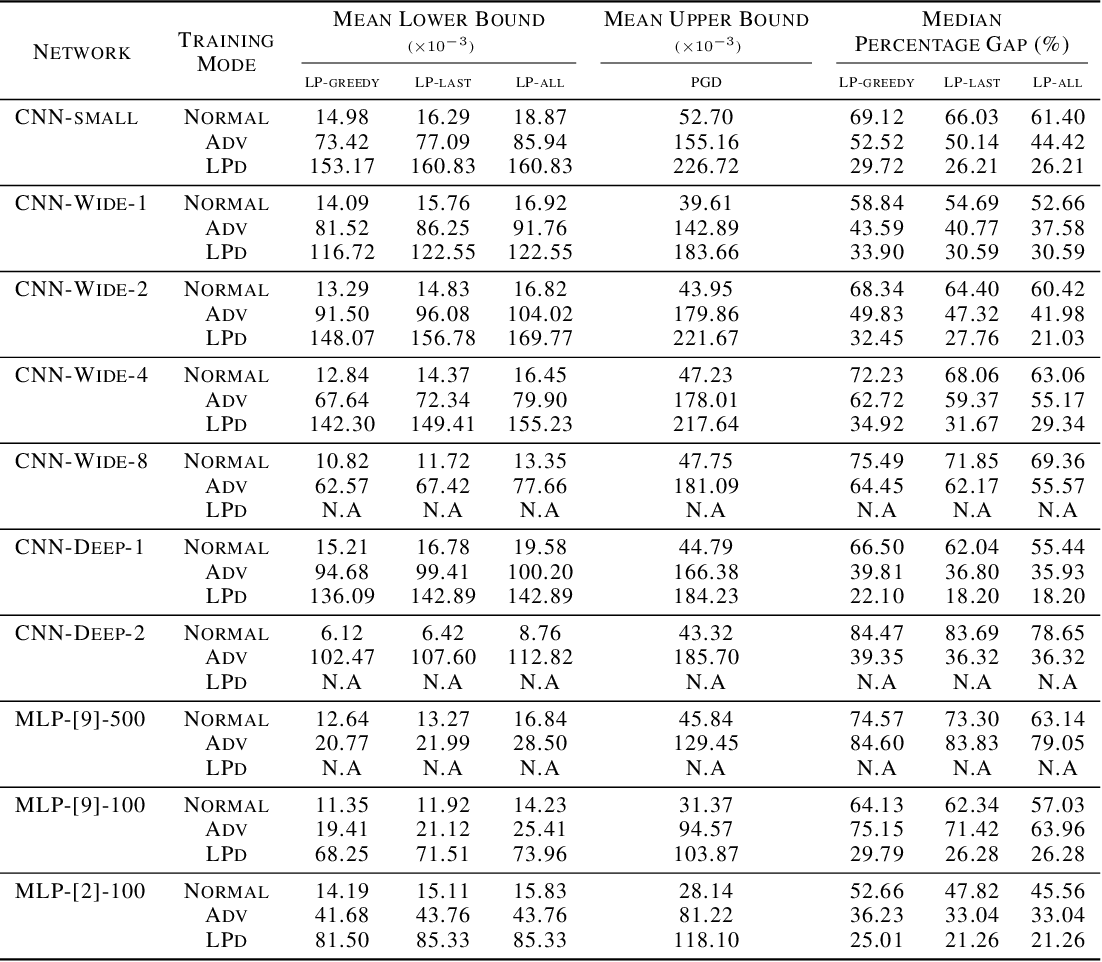Verification of neural networks enables us to gauge their robustness against adversarial attacks. Verification algorithms fall into two categories: exact verifiers that run in exponential time and relaxed verifiers that are efficient but incomplete. In this paper, we unify all existing LP-relaxed verifiers, to the best of our knowledge, under a general convex relaxation framework. This framework works for neural networks with diverse architectures and nonlinearities and covers both primal and dual views of robustness verification. We further prove strong duality between the primal and dual problems under very mild conditions. Next, we perform large-scale experiments, amounting to more than 22 CPU-years, to obtain exact solution to the convex-relaxed problem that is optimal within our framework for ReLU networks. We find the exact solution does not significantly improve upon the gap between PGD and existing relaxed verifiers for various networks trained normally or robustly on MNIST and CIFAR datasets. Our results suggest there is an inherent barrier to tight verification for the large class of methods captured by our framework. We discuss possible causes of this barrier and potential future directions for bypassing it.

Click to Read Paper and Get Code